Question

1. Using appropriate methods, solve the following differential equations analytically (by handi.e. without using any software) to obtain the complete solutions. Clearly show all steps, boxthe final answer.

(a) Using the substitution (or change of variable)u = 1 - 2y, solve the differential equation

(2 x-4 y+5) y^{\prime}+x-2 y+3=0

Show all steps of the substitution and finally express your solution in terms of y and ronly.

) Newton's law of cooling leads to the differential equation

\frac{d T}{d t}=-k\left(T-T_{1}\right)

where T(t) is the temperature of a body placed in a medium which is kept at a constant temperature T. Solve the equation assuming the initial temperature of the body to beT(0) = To. Show all steps.

Using the substitution (or change of variable) u = 1/y, solve the differential equation

y^{\prime}+y / x=y^{2} / x

Show all steps of the substitution and finally express your solution in terms of y and aonly.

) Solve the equation to obtain the general solution using: (I) integrating factors, (ii)method of undetermined coefficients, (iii) variation of parameters.

y' +y = e

e) An extended object falling downward is known to experience resistive force of the air(called drag). We assume the magnitude of this force be proportional to the speed v.Using Newton's second law, we get,

m \dot{v}=-k v-m g

where idv/dt and g is gravitational acceleration at the surface of the earth. Solve this equation assuming v(0) = v: Then integrating the result, find y(t), the distance at time t measured from the starting point yo = y(0).Verified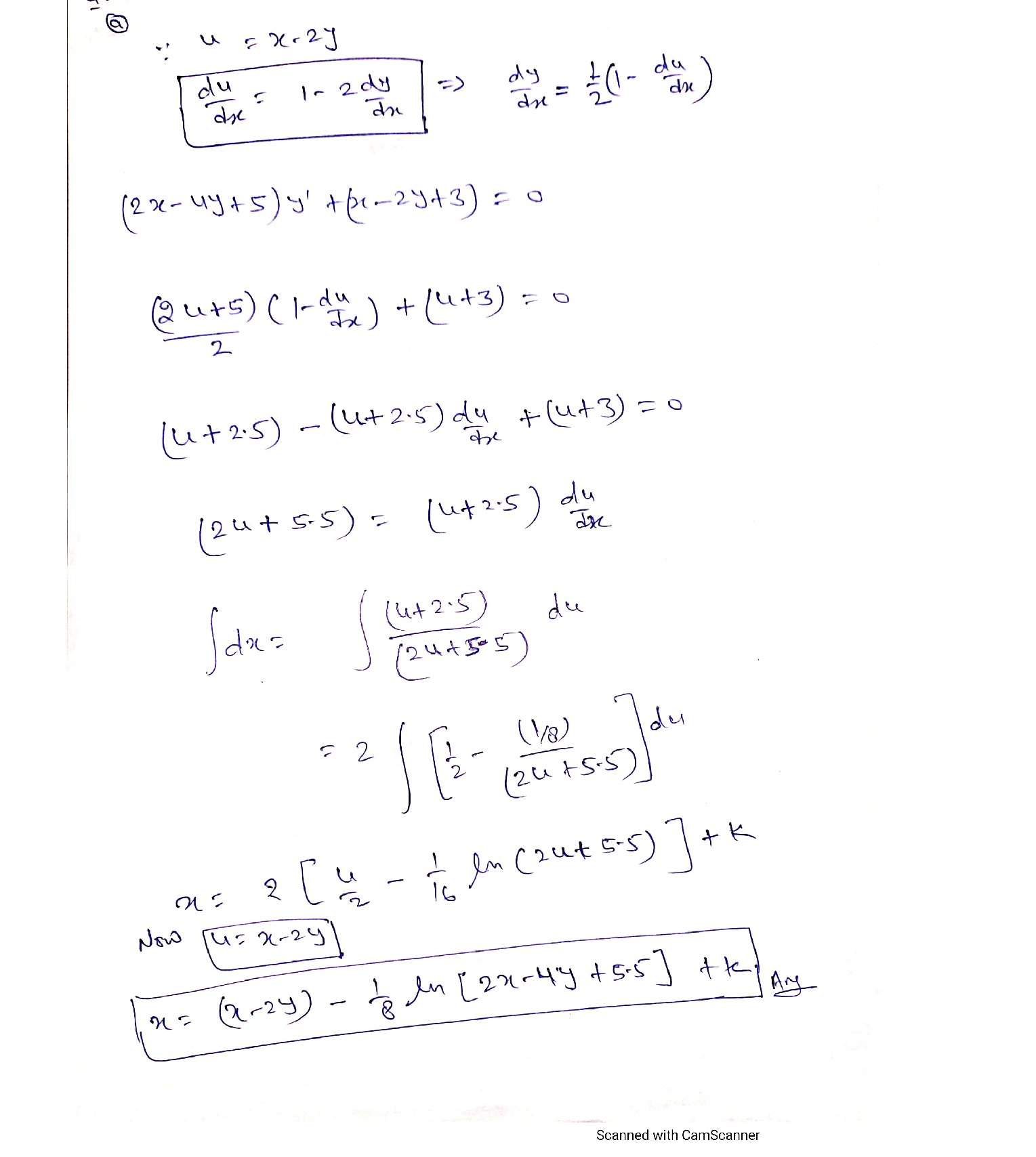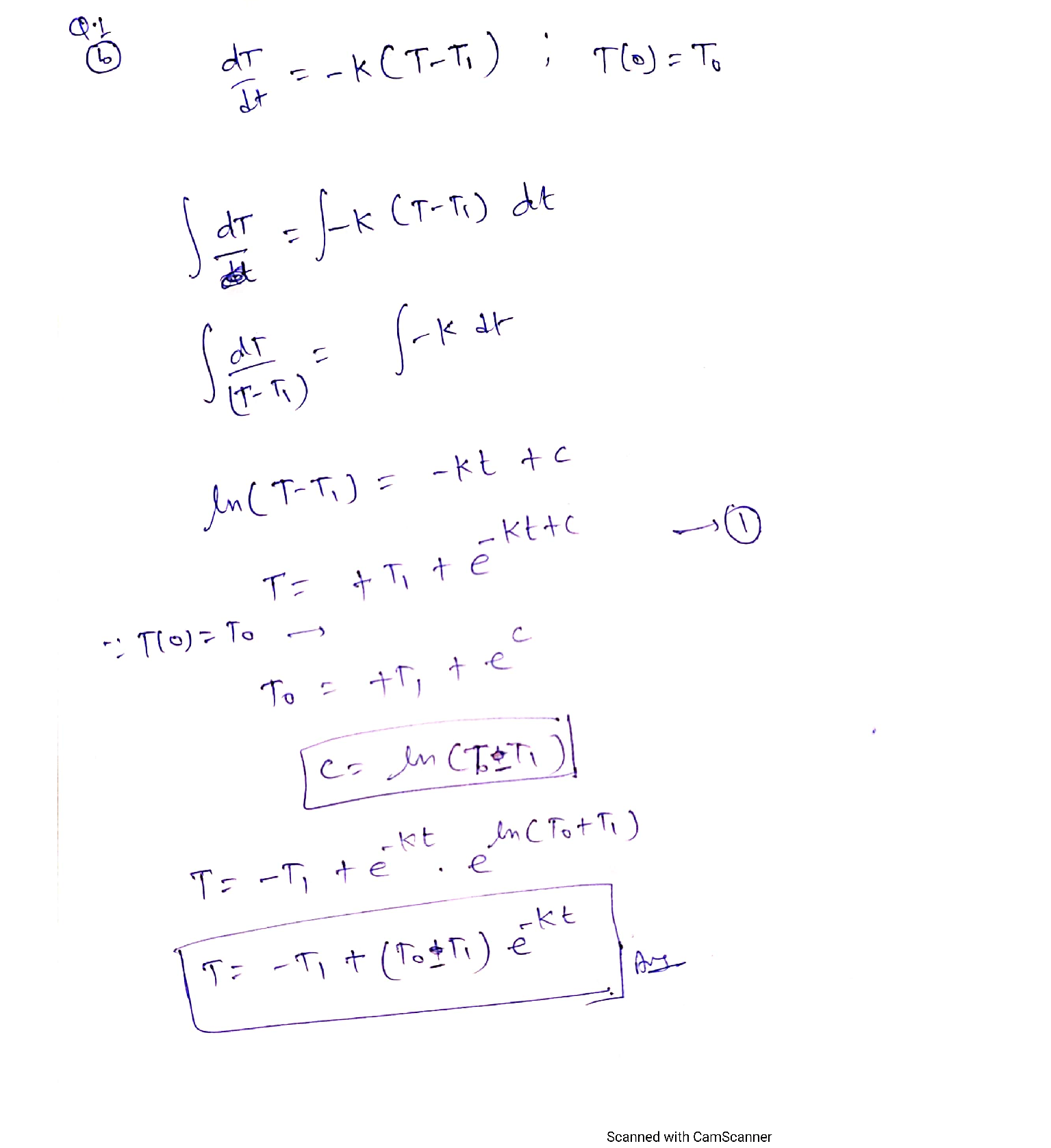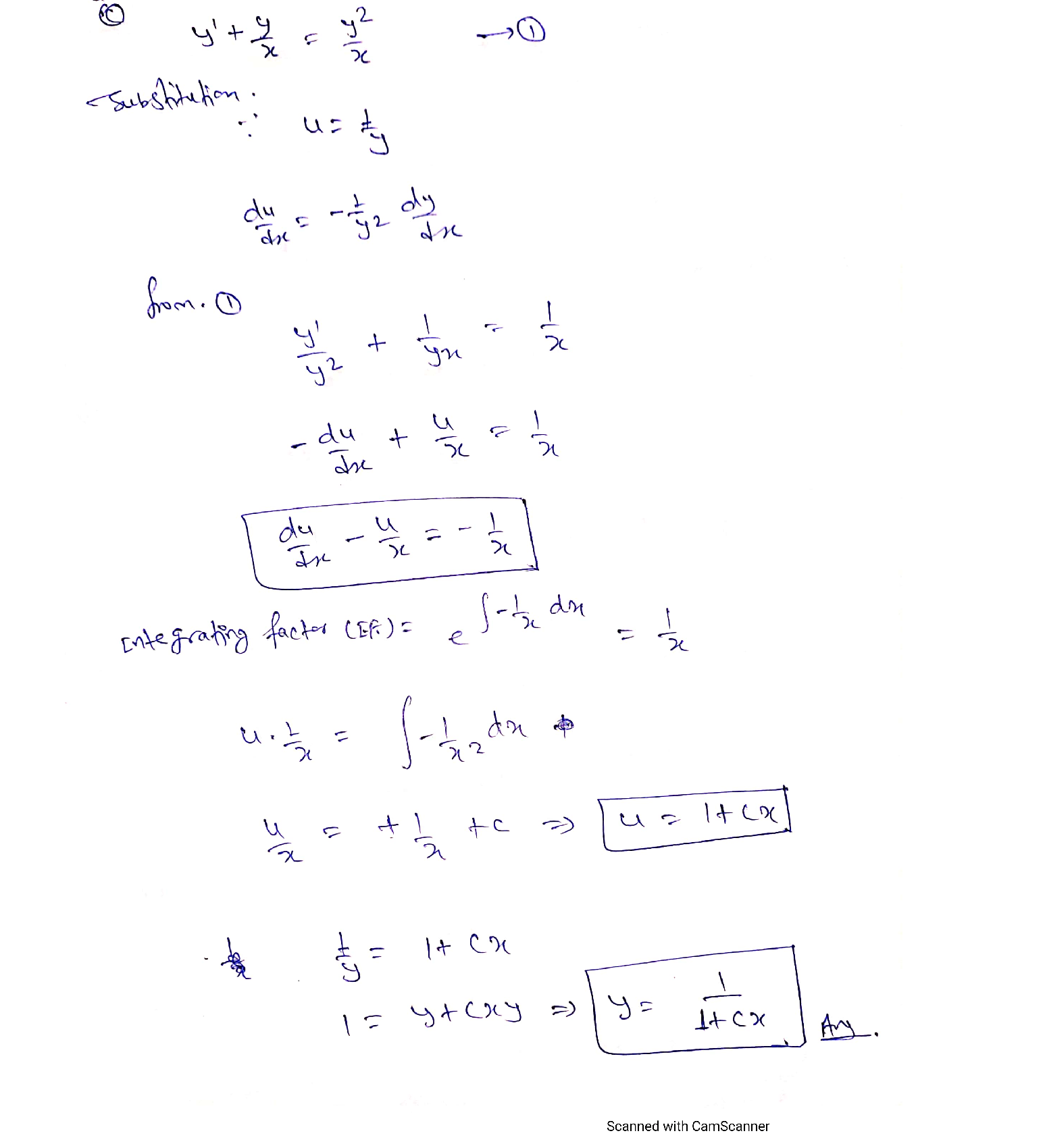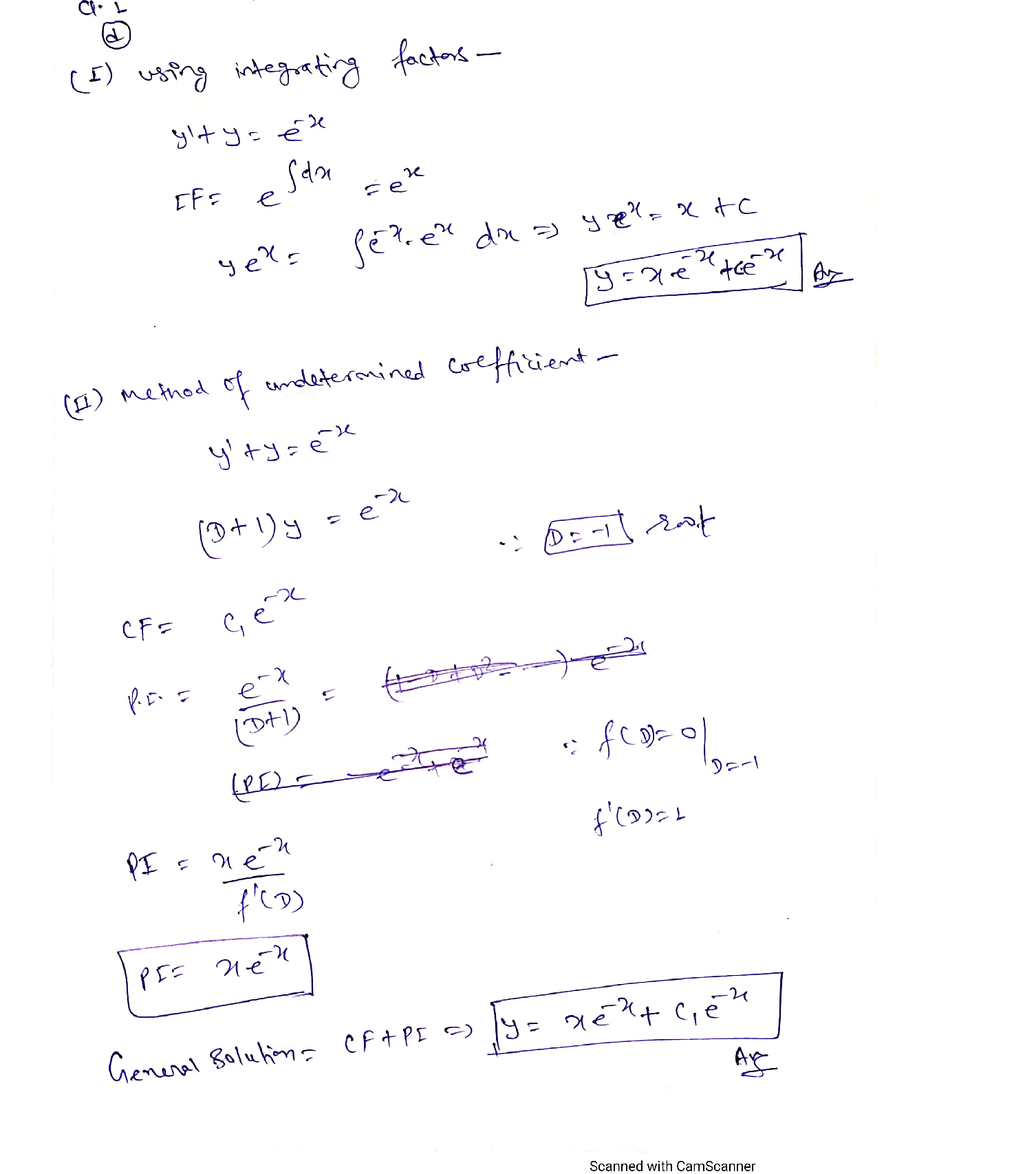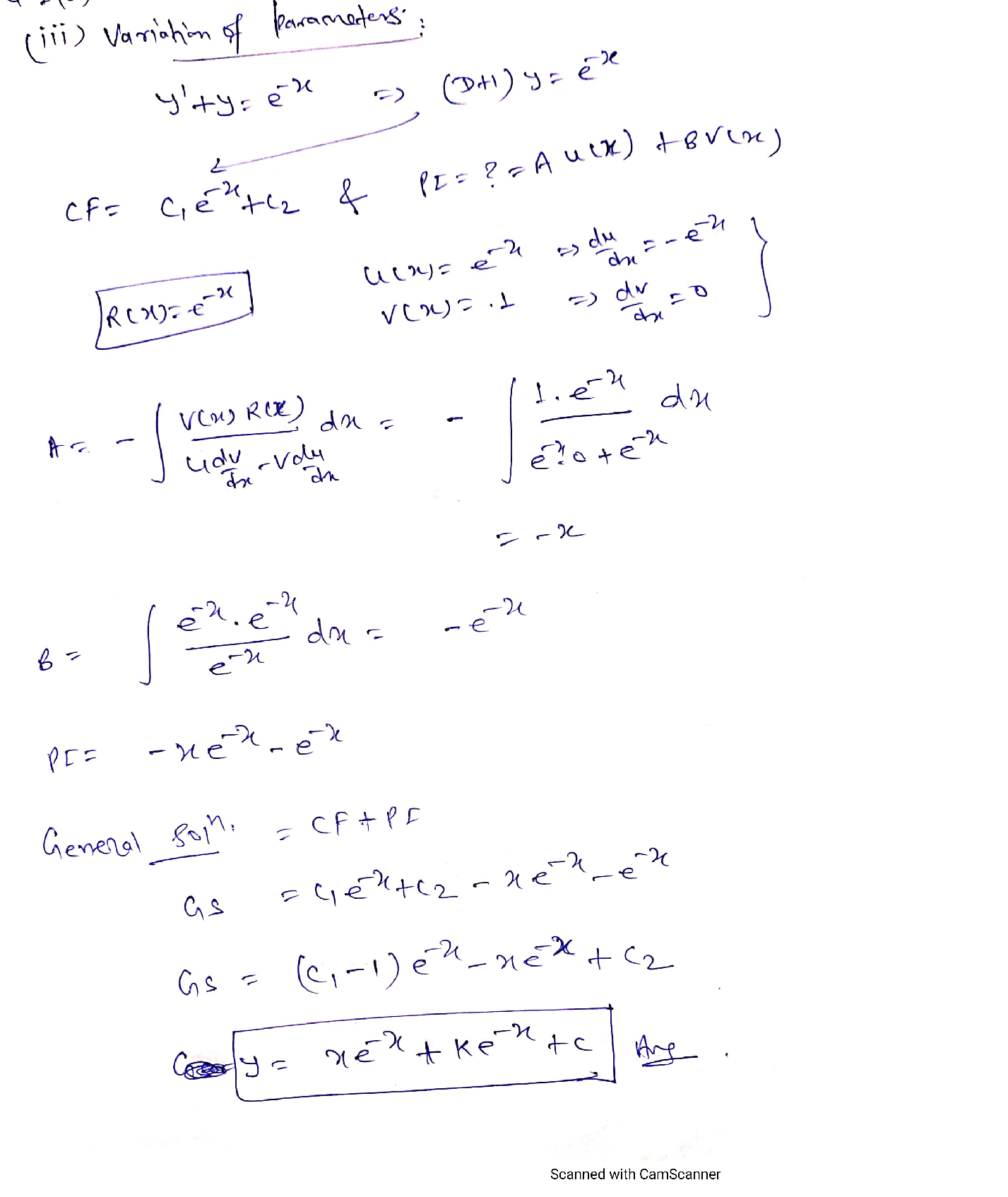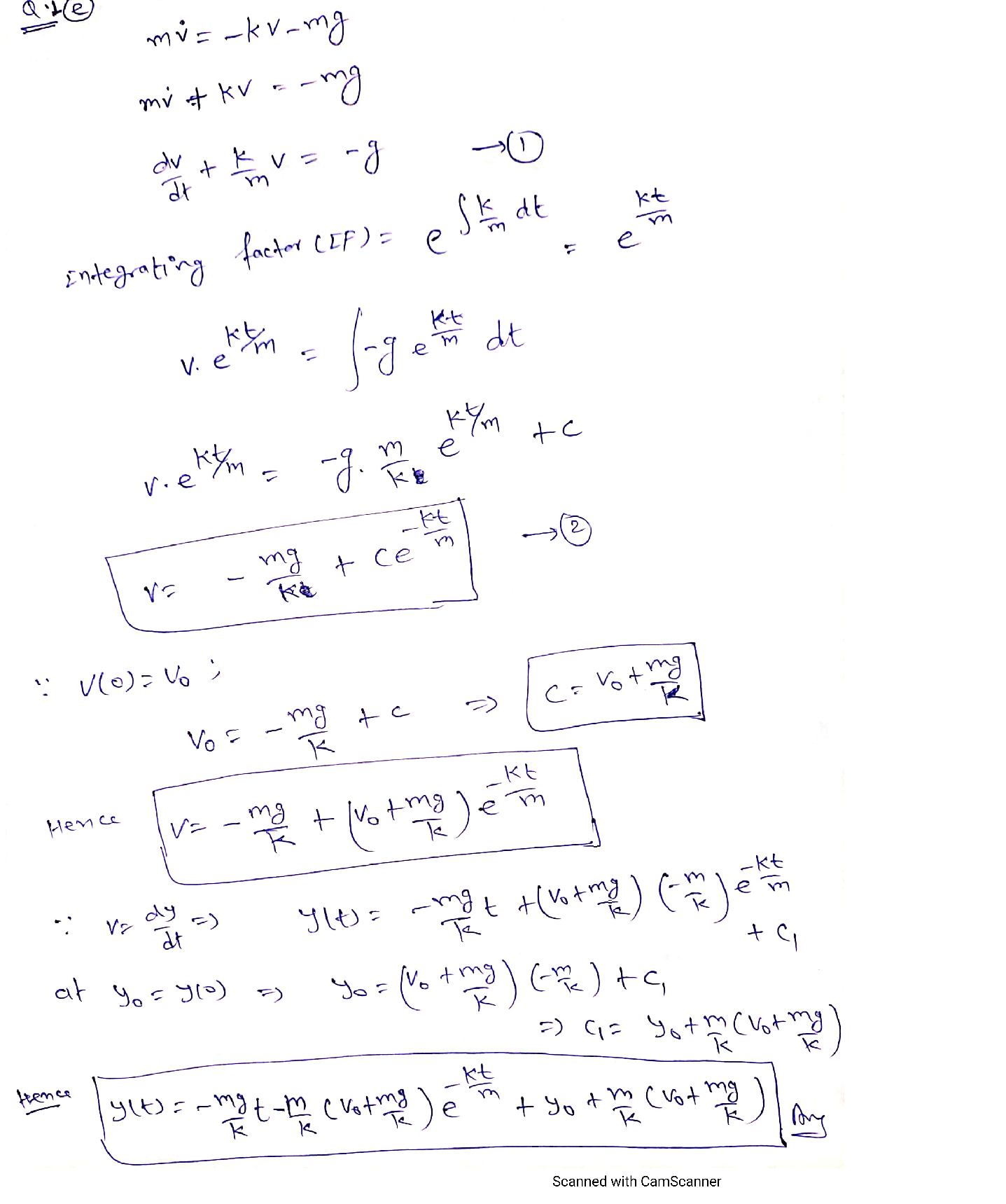### Question 44046During the summer months, British Columbia experiences numerous forest fires. You are working as a fire ranger in the interior of British Columbia and you spot a fire that is 15 km away from your tower. Your friend is working at another tower that is located 20 km from you at an angle of 21°. She can also see the fire.
b) Your friend decides to dispatch a water plane to the fire. Your station is not equipped with waterplanes. At what angle should the plane fly to target the fire accurately? (AP3)
Your friend decides to dispatch a water plane to the fire. Your station is not equipped with water planes. At what angle should the plane fly to target the fire accurately? (AP3)

### Question 44045As a computer engineer, you are always looking at ways to improve the computing experience. As part of your final paper to submit for your Master's Degree, you do some research about how far computing has come over the years.
You make some interesting discoveries. Dating back to 1940, you find out that one of the original large computers was able to perform about 100 operations per second. After examining the data,you see that the speed of computers has multiplied 5-fold about every 7 years.
a) Some of the data is missing. You know that some super computers can now process4 882 812 500 operations per second. How many years have gone by to reach this number of operations? What year would this have occurred in? Show your solution algebraically! (AP5)
b) Do you think this is a realistic model? What limitation(s) may arise with this model? (CM3)

### Question 43374Find the distance between the parallel planes P: 5x - 3y + z=1 and P: 10x-6y +2z=4. Enter an exact answer, do not use decimal approximation.

### Question 43373Find an equation for the plane through the point (3, 2,-5) and parallel to the plane defined by5x- 4y + 4z = 12.
1=5(x-3)-4(y+5)+10(z-2)
0=-4(x+3)+5(y-2)+4(z+5)
1=5(x-3)-4(y-2)+4(z+5)
0=5(x-3)-4(y-2)+4(z+5)

### Question 43372\text { Find the plane containing the three points } P(3,-2,1), Q(1,-3,0) \text { and } R(3,-5,5) \text {. }
0=-7(x+3)+8(y-2)+6(z+1)
0=-7(x-3)+8(y+2)+6(z-1)
0=-7(x-3)-8(y+2)+6(z-1)
1=-7(x-3)+8(y+2)+6(z-1)

### Question 43371Find the directions of maximum and minimum change of f at the given point, and the values of the maximum and minimum rates of change.
f(x, y)=5 y^{2} e^{7 x},(4,-3)
\text { The maximum change is } 15 e^{28} \sqrt{445} ; \text { in the direction }\left(-315 e^{28}, 30 e^{28}\right)
\text { The minimum change is }-15 e^{28} \sqrt{445} ; \text { in the direction }\left(315 e^{28},-30 e^{28}\right) \text {. }
\text { The maximum change is } 15 e^{-28} \sqrt{445} ; \text { in the direction }\left(-315 e^{-28}, 30 e^{-28}\right)
\text { The minimum change is }-15 e^{-28} \sqrt{445} ; \text { in the direction }\left(315 e^{-28},-30 e^{-28}\right)
\text { The maximum change is } 15 e^{28} \sqrt{445} ; \text { in the direction }\left(315 e^{28},-30 e^{28}\right\rangle
\text { The minimum change is }-15 e^{28} \sqrt{445} ; \text { in the direction }\left\{-315 e^{28}, 30 e^{28}\right)
\text { The maximum change is } 15 e^{-28} \sqrt{445} ; \text { in the direction }\left(315 e^{-28},-30 e^{-28}\right)
\text { The minimum change is }-15 e^{-28} \sqrt{445} \text {; in the direction }\left(-315 e^{-28}, 30 e^{-28}\right\rangle

### Question 43370Select the description and the sketch of the domain of the function.
f(x, y)=\frac{4 x y}{\left(y-x^{2}\right)}

### Question 43369\text { Find an equation of the line passing through the points } P(3,4,-2) \text { and } Q(5,-4,3) \text {. }
\frac{x+3}{2}=\frac{y+4}{-8}=\frac{z-2}{5}
\frac{x+4}{-4}=\frac{y+4}{5}=\frac{z-3}{3}
\frac{x-3}{2}=\frac{y-4}{-8}=\frac{z+2}{5}
x-3=y-4=z+2

### Question 43368Round each component to four decimal places, if necessary.
\text { Find the gradient of } f(x, y)=3 e^{5 x d y}+2 x \text { at }(-1,-1)
\nabla f(-1,-1) \approx

### Question 43367\text { Find the gradient of the function } f(x, y)=x^{2}+4 x y^{2}-y^{7}

### Submit query